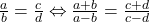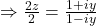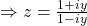# MCQ Complex Numbers and Quadratic Equations

The value ofis equal to
(A) 4
(B) 6
(C) 8
(D) 2

Explanation:
We have[by De-Moivre’s theorem]The solution of the equation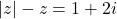is
(A)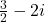(B)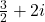(C)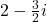(D)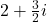Explanation:
Let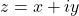Comparing real and imaginary parts
we have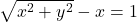and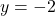Now substitute the value of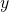inwe get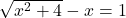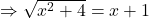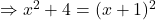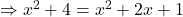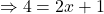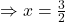If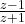is purely imaginary, then
(A)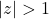(B)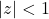(C)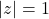(D) None of these

Let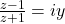, where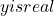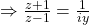As we know by componendo and dividendo that